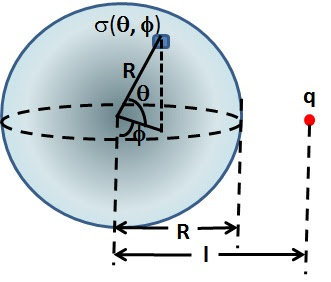## Saturday, November 20, 2010

### Irodov Problem 3.63Couple of concepts need explanation here first before the problem can be solved. So I shall first provide some explanations and then proceed to solve the problem.

All charges will reside only on the surface of the solid spherical conductor
The basic thing to understand in this problem is that electrons flow freely within conductors. As described in problem 3.54, the electric potential at all points in a conducting body should be equal. Otherwise since in a conductor charges are free flowing, the charges would freely flow and redistribute themselves until potential is equalized. Since there is no potential difference between any two points within the conducting body, there cannot be any electric field inside the solid conducting sphere. Since there is not electric filed inside the solid sphere, there cannot be any charge distribution inside the sphere (from Gauss law if there where any charge distribution inside the sphere, there would be an electric field). This leads to an inescapable conclusion that charges in the conducting sphere will only reside on the surface of the sphere. In other there will only be a surface charge density in the solid sphere.

The total charge in the sphere is zero
Since the sphere had no charge of its own to being with, and charges have only redistributed themselves, the net charge of the sphere must be zero. In other words if the surface charge density of the sphere at a location, R being the radius of the sphere, is(R is ignored since all charge resides at the surface only), then,

Potential of the sphere
Since the entire conducting sphere is at the same potential, determing the potential at any one point within the sphere is sufficient to determine the potental at any point in the sphere. It turns out that it the easiest to determine the potential at the center of the sphere. The total potential at the center of the sphere is given by, the potential due to i) the charge q outside the sphere and ii) due to the surface charge density induced in the surface of the conducting sphere.
This is given by,